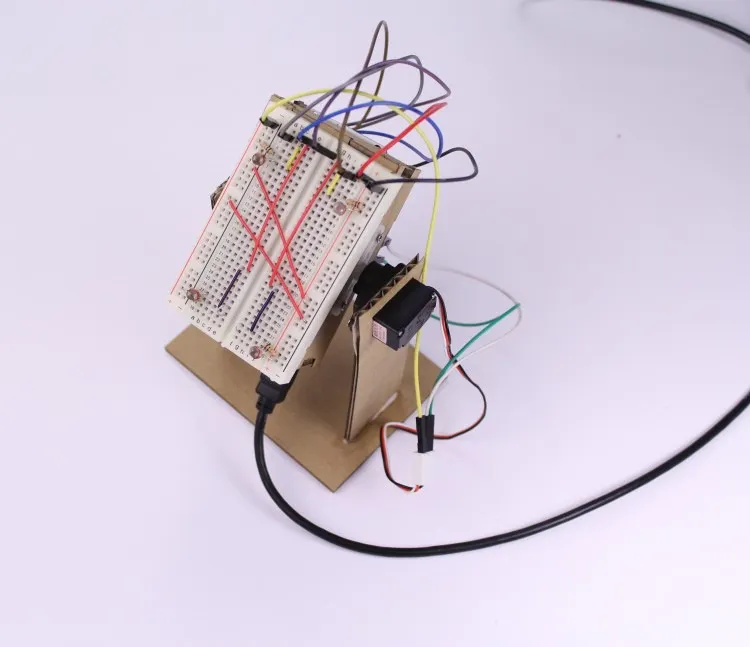Project tutorial# A Simple Light Follower © CC BY-NC-SA

This lesson aims to show how to make a simple light follower made of cardboard using a microservo.

• 6,233 views
• 1 comment
• 9 respects

## Components and suppliesArduino UNO & Genuino UNO
×1
×1
 10 jumper wires 200mm male
×1
 5 LDR
×1
 10 10k 1/4w resistor
×1
 Analog 180° Micro Servo
×1
 4mm cardboard
×1

## Apps and online services

### 1. How does it Work?

The choice to go up or down in order to follow the light is done in a very simple way. There are four LDR two up and two down. Arduino reads the analog value of all LDR, if the average of the two upper values is greater than the two lower the light is up so the angle of the microservo is incremented otherwise decremented.

### 2. A Little Clarification

Since the average is not a fixed number, as you can see in the code, a threshold is used. In this way the angle is changed if and only if the two averages are widely different.

``````const int threshold = 50;
[.....]
down_average = (A0_val + A1_val)/2;
up_average = (A2_val + A3_val)/2;
diff = abs(up_average-down_average);
if ((up_average > down_average) && (diff > threshold))
{
if (val < 180) //if different from max val
{
val++;
myservo.write(val);
}
}
if((down_average > up_average) && (diff > threshold))
{
if (val > 0) //if different from min val
{
val--;
myservo.write(val);
}
}
``````

### 3. The Circuit

Make the circuit below!

### 4. Print & Cut

Download the attached file, print it, glue it on the cardboard and cut all the pieces for yours light follower. You should obtain something like this.

### 5. Mount the Base

Mount the breadboard base in this way. Glue it if necessary.

### 6. Mount the Servo

Mount the servo like below. Screw it for more stability.

### 7. Mount the Bracket

Mount the bracket in this way.

### 8. Mount the Servo Gear

Mount the servo gear. Screw it in this way.

### 9. Put all Together

Put all together. You should obtain something like this

### 11. Screw Arduino

Screw Arduino on the back of the base.

## Code

##### Light Follower codeArduino
```/*
A simple light follower

*/
#include <Servo.h>
Servo myservo;
int up_average;
int down_average;
int A0_val;
int A1_val;
int A2_val;
int A3_val;
int val = 90;
int diff;
const int threshold = 50;
void setup() {
pinMode(A0, INPUT);
pinMode(A1, INPUT);
pinMode(A2, INPUT);
pinMode(A3, INPUT);
myservo.attach(11);
myservo.write(val);
}
void loop() {

down_average = (A0_val + A1_val)/2; //the sum of the two lower sensors /2
up_average = (A2_val + A3_val)/2;	  //the sum of the two upper sensors /2
diff = abs(up_average-down_average); //checking the difference between the two averages
if ((up_average > down_average) && (diff > threshold))
{
if (val < 180) //if different from max val
{
val++;
myservo.write(val);
}
}
if((down_average > up_average) && (diff > threshold))
{
if (val > 0) //if different from min val
{
val--;
myservo.write(val);
}
}
delay(25);
}
```

## Custom parts and enclosures

LightFollower.dxf

## SchematicsJuly 8, 2016

#### Members who respect this project

See similar projects
you might like

#### Simple Line Follower Robot

Project tutorial by WolfxPac

• 3,437 views
• 10 respects

#### Simple Programmable Robotic Arm

Project showcase by Ryan Chan

• 85,714 views
• 219 respects

#### Controlling LED light intensity using smartphone light senso

Project tutorial by Nikola Travis

• 2,670 views
• 1 respect

#### PID Control Line Follower Robot

Project tutorial by Team KittenBot

• 24,004 views
• 36 respects

#### Line Follower Robot Using Arduino & 1Sheeld

Project tutorial by Ashraf Nabil

• 3,333 views